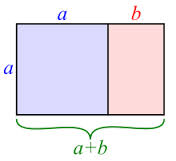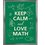# Golden ratio #ShoMinamotoProblemsShoMinamoto: Before I start this discussion let me Introduce myself. My name is Sho Minamoto otherwise known as Pi face. And this is me introducing an "zetta" outstanding number which they call GOLDEN RATIO.

Golden Ratio The golden ratio also is called the golden mean or golden section (symbol is the Greek letter "phi" shown at left). It is a special number approximately equal to 1.618., It appears many times in geometry, art, architecture and other areas.

The Idea behind it

We find the golden ratio when we divide a line into two parts so that:

The longer part divided by the smaller part

Is also equal to

The whole length divided by the longer part

[a/b]=[(a+b)/a]

And it gets [(1+sqrt(5))/2]

And it keeps on getting interesting when we relate it to geometric figures.

Sho Minamoto: So do you find it interesting ? I find it interesting. try watching https://www.khanacademy.org/math/geometry/intro_euclid/v/the-golden-ratio6 years, 7 months ago

This discussion board is a place to discuss our Daily Challenges and the math and science related to those challenges. Explanations are more than just a solution — they should explain the steps and thinking strategies that you used to obtain the solution. Comments should further the discussion of math and science.

When posting on Brilliant:

• Use the emojis to react to an explanation, whether you're congratulating a job well done , or just really confused .
• Ask specific questions about the challenge or the steps in somebody's explanation. Well-posed questions can add a lot to the discussion, but posting "I don't understand!" doesn't help anyone.
• Try to contribute something new to the discussion, whether it is an extension, generalization or other idea related to the challenge.

MarkdownAppears as
*italics* or _italics_ italics
**bold** or __bold__ bold
- bulleted- list
• bulleted
• list
1. numbered2. list
1. numbered
2. list
Note: you must add a full line of space before and after lists for them to show up correctly
paragraph 1paragraph 2

paragraph 1

paragraph 2

[example link](https://brilliant.org)example link
> This is a quote
This is a quote
    # I indented these lines
# 4 spaces, and now they show
# up as a code block.

print "hello world"
# I indented these lines
# 4 spaces, and now they show
# up as a code block.

print "hello world"
MathAppears as
Remember to wrap math in $$ ... $$ or $ ... $ to ensure proper formatting.
2 \times 3 $2 \times 3$
2^{34} $2^{34}$
a_{i-1} $a_{i-1}$
\frac{2}{3} $\frac{2}{3}$
\sqrt{2} $\sqrt{2}$
\sum_{i=1}^3 $\sum_{i=1}^3$
\sin \theta $\sin \theta$
\boxed{123} $\boxed{123}$